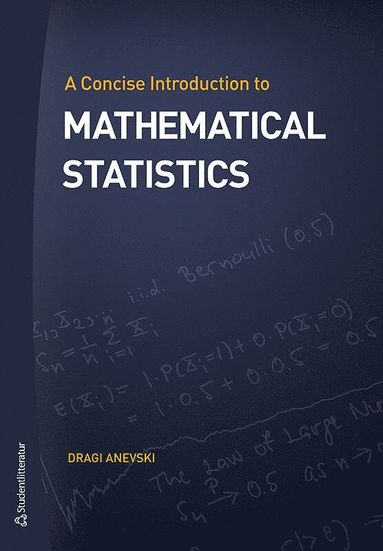Vetenskap & teknik

# A concise introduction to mathematical statistics

## Dragi Anevski

509:-

Funktionen begränsas av dina webbläsarinställningar (t.ex. privat läge).

Fri frakt för medlemmar vid köp för minst 149:-

• 420 sidor
• 2017
This book gives a thorough introduction to mathematical statistics. The text is unique as an introductory text, mainly by the use of the Riemann-Stieltjes integral. This enables a unified treatment of basic concepts in probability and inference theory, in a mathematically rigorous manner, without the use of measure theory. The approach differentiates this book from other introductory texts, where one does not give a unified approach to basic concepts, as well as from advanced texts, where one does give a unified approach relying on advanced mathematics. The treatment of probability theory differs from comparable books in that one discusses basic concepts rigorously but without the use of Lebesgue integration. Thereby it allows one to concentrate on the basic concepts of mathematical statistics, without sacrifice of mathematical stringency. The approach also enables a concise definition of the plug-in estimator in inference theory. Arguably, the plug-in estimator is the most natural and intuitive estimator possible. The introduction of it is however mathematically advanced, and typically covered in PhD level texts. Using the Riemann-Stieltjes integral the introduction of it becomes elementary. The book is intended for students at the Faculty of Science and Faculty of Engineering that have taken a full year of basic mathematics courses, including real analysis and linear algebra.
• Författare: Dragi Anevski